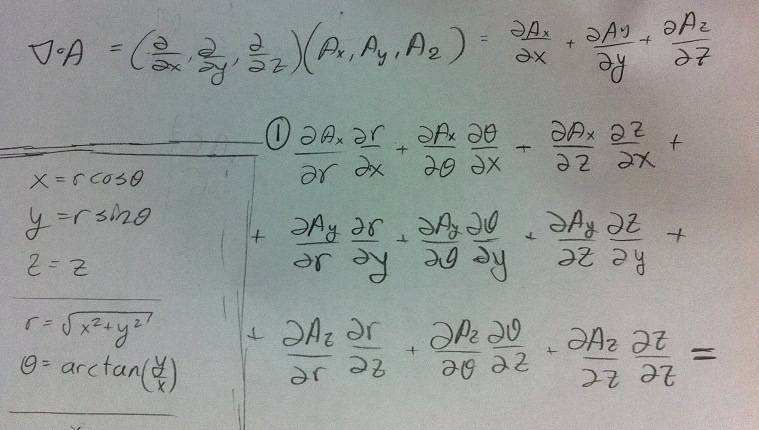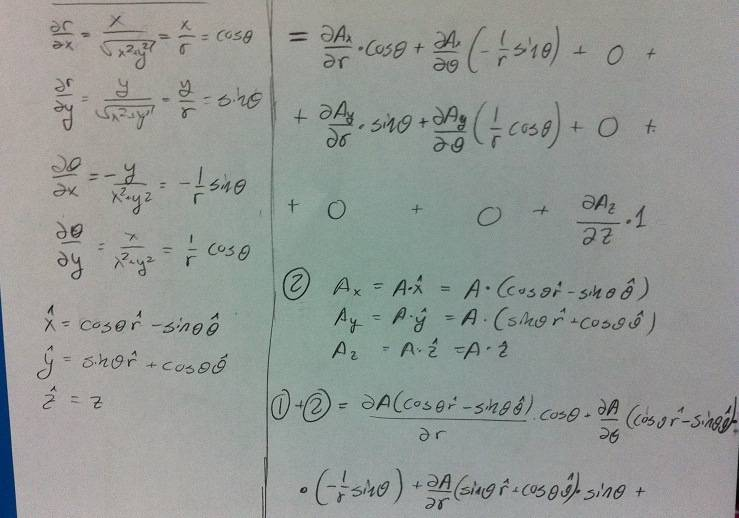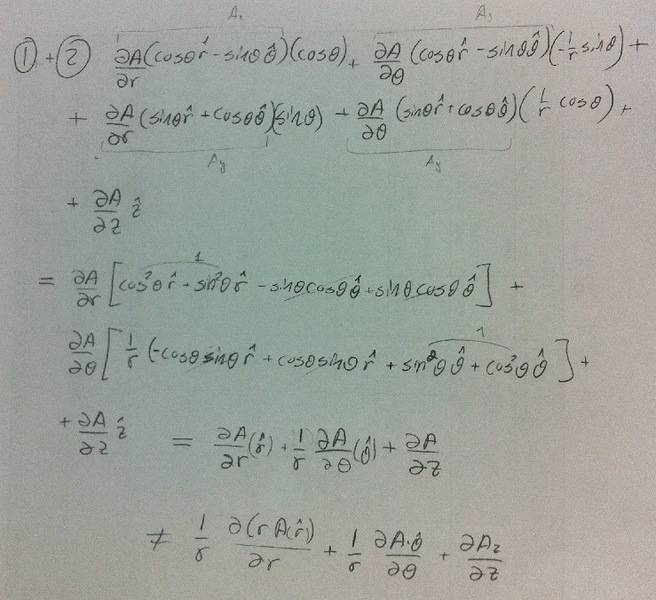# Switch the divergence coordinate system

• oronanschel

## Homework Statement

i have the divergence in the (x,y,z) Cartesian as $$\frac{dA_x}{dx}+\frac{dA_y}{dy}+\frac{dA_z}{dz}$$

and the assignment is to transfer it to cylindrical system $$(r,{\phi},z)$$, by any way i choose.

## Homework Equations

tried with the chain rule, but i am doing something wrong, though
i get the gradient in the cylindrical system

It is impossible to tell you what, if anything, you are doing wrong if we cannot see what you did!

look at 1 then 2

then there is 1+2

in the left side there are some derivatives , and variables
transformation to explain